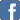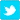# The Learning Experiment - Organizing and Memorizing (Part 3) - Using Mole Concept as Example

Posted on the 07 December 2016 by Ewong0117
In this post, I will be using the chapter on mole concept as an example of how to organize information for easy memorization.
If you take a closer look back at the post on mole concept formulae, you would notice that I have arranged the formulae according to the states (i.e. solutions, gases, for all states). This is an example of how you can organize information for easy memorization.
As such, you should always remember that this formula is always application for all situations, whether solids, liquids, gases, solutions:
• mole = mass/ (molar mass)
• mole = (number of particles) / (Avogadro's constant)

When you see solutions (aqueous state), the following applies:
• mole = concentration in mol/dm3 x volume in dm3
• mass = concentration in g/dm3 x volume in dm3

When you see gases, the following apply:
• mole = volume of gas / molar volume

Now, you have three groups of information, and each group has 1 to 2 formula(e), making memorization and recalling them easier.
Related Post:
-
The Learning Experiment - Organization and Memorization (Part 2)
- Mole Concept - Learning from Example
- Mole Concept Formulae
Related Questions:
- Quizzes and Tests on  Mole Concept